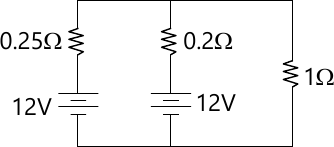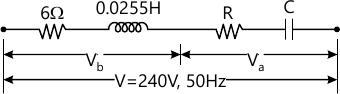MORE IN Basic Electrical Engg.
VTU First Year Engineering (P Cycle) (Semester 1)
Basic Electrical Engg.
January 2012
Total marks: --
Total time: --
INSTRUCTIONS
(1) Assume appropriate data and state your reasons
(2) Marks are given to the right of every question
(3) Draw neat diagrams wherever necessary

Choose the correct answer for the following :-
1 (a) (i) Two resistor R1 and R2 give combined resistance of 4.5 Ω when in series and I Ω when in parallel, the resistance are
(A) 2 Ω and 2.5 Ω
(B) 1 Ω and 3.5 Ω
(C) 1.5 Ω and 3 Ω
(D) 4 Ω and 0.5 Ω
1 M
1 (a) (ii) Kirchoff's voltage law applies to circuit with
(A) linear elements only
(B) non-linear elements only
(C) linear, non-linear, active and passive elements
(D) linear, non-linear, active, passive, tine varying as well as time invariant elements.
1 M
1 (a) (iii) Energy consumed by a heater of rating 1000W by operating it for a period of 2 hrs will be
(A) 1 kWh
(B) 2 kWh
(C) 2.5 kWh
(D) 4 kWh
1 M
1 (a) (iv) A practical voltage source is represented by
(A) a resistance in parallel with an ideal voltage source
(B) a resistance in series with an ideal current source
(C) a resistance in series with an ideal voltage source
(D) None of the above.
1 M
1 (b) For the circuit shown in fig. Q1 (b), find the current supplied by each battery and power disspated in Ω resistor.6 M
1 (c) Explain the Fleming's rules and their use in electromagnetism.
6 M
1 (d) A solenoid 1m in length and 10 cm in diameter has 5000 turns. Calculate the inductance and energy stored in the magnetic field when a current of 2A flows in the solenoid.
4 M

Choose the correct answer for the following :-
2 (a) (i) Definition of root-mean square value is
(A) Square root of area under the square curve over half cycle to length of base over half cycle
(B) Average value by √2
(C) Ratio of maximum value to average value
(D) None of the above
1 M
2 (a) (ii) The equation of an alternating current is i=42.42 Sin 628t. The effective value will be
(A) 27A
(B) 30A
(C) 2.7A
(D) 3A
1 M
2 (a) (iii) The maximum and minimum values of power factor can be
(A) +1 and -1
(B) +1 and -5
(C) +1 and 0
(D) +5 and -5
1 M
2 (a) (iv) By adding more resistance to an RC circuit
(A) the real power increases
(B) the real power decreases
(C) the power factor decreases
(D) the phase difference increases
1 M
2 (b) Draw the phasor diagram for RL series circuit and derive the expression of real power.
6 M
2 (c) For the circuit shown in fig. Q2(c), find the value of R and C so that vb=3 va and vb and va are in quadrature.6 M
2 (d) Two impedances z1=(10 + j15)Ω and z2=(5 - j8)Ω are connected in parallel across a voltage source. If the total current drawn is 10A, calculate currents in z1 and z2 and power factor of the circuit.
4 M

Choose the correct answer for the following :-
3 (a) (i) The sum of the two-wattmeters readings in a 3 phase balanced system is
(A) VphIphCos ϕ
(B) 3 VLILCosϕ
(C) ϕ3 VLILCosϕ
(D) None of these
1 M
3 (a) (ii) The rated voltage of a 3 phase system is given as
(A) rms phase voltage
(B) peak phase voltage
(C) rms line-to-line voltage
(D) peak line-to-line voltage
1 M
3 (a) (iii) A 3 phase star connected load consumers P watts of power from a 400V supply. If the same balanced load is connected in delta across that same supply, then power consumption is
(A) 3 P
(B) √3 P
(C) P/3
(D) p
1 M
3 (a) (iv) The phase sequence RBY denotes that
(A) emf of phase-B lags that of phase-R by 120°
(B) emf of phase-B that of phase-R by 120°
(C) Both A and B are connected
(D) None of these
1 M
3 (b) Derive the relationship between line and phase values of balanced star and delta connected load with balanced supply.
8 M
3 (c) A 3-phase delta connected load consumes a power of 60 kW taking a lagging current of 200A at a line voltage of 400V, 50Hz. Find the parameters of each phase. What would be the power consumed, if the load were connected in star?
8 M

Choose the correct answer for the following :-
4 (a) (i) The moving coil in a dynamometer wattmeter is connected
(A) in series with the fixed coil
(B) across the supply
(C) in series with the load
1 M
4 (a) (ii) The voltage coil of a single phase energy meter
(A) is highly resistive
(B) is highly inductive
(C) is highly capacitive
(D) has a phase angle equal to load p.f. angle.
1 M
4 (a) (iii) The meter constant of energy meter is given by
(A) rev./kW
(B) rev./watt
(C) rev./kWh
(D) rev./kVA
1 M
4 (a) (iv) The primary function of a fuse is to
(A) protect the appliance
(B) open the circuit
(C) prevetnt excessive current
(D) protect the line
1 M
4 (b) Explain the principle of operation of dynamometer type wattmeter.
6 M
4 (c) With diagrams, explain the three-way control of a lamp.
4 M
4 (d) With a neat diagram, explain the plate earthing.
6 M

Choose the correct answer for the following :-
5 (a) (i) The function of a commutator in d.c. generator is
(A) to collect current from conductors
(B) to change d.c. to a.c.
(C) to conduct the current to brushes
(D) to change a.c. to d.c.
1 M
5 (a) (ii) The current drawn by armature of a d.c. motor is
(A) V/Ra
(B) Eb/Ra
(C) (V-Eb)/Ra
(D) (Eb-V)/Ra
1 M
5 (a) (iii) The speed of a series motor at no-load is
(A) zero
(B) 1500 rpm
(C) 3000 rpm
(D) infinity
1 M
5 (a) (iv) The torque of a shunt motor is propertional to
(A) armature current
(B) applied voltage
(C) square of the armature current
(D) none of these
1 M
5 (b) What are the functions of yoke, armature, poles and brushes in a d.c. generator?
4 M
5 (c) Derive the expression for armature torque developed in a d.c. motor.
6 M
5 (d) A 100 kW belt driven shunt generator running at 300 rpm on 220V bus-bars, continues to run as a motor when the belt breaks, then taking 10 kW. What will be its speed? Given Ra=0.025 Ω, Rsh=60?, BCD=1V per brush, and ARD=0
6 M

Choose the correct answer for the following :-
6 (a) (i) The magnitude of mutual flux in a transformer is
1 M
6 (a) (ii) Transformer cores are laminated in order to
(A) Simplify its construction
(B) minimize eddy current loss
(B) (C) reduce cost
(D) reduce hystresis loss
1 M
6 (a) (iii) The transformation ratio of a transformer is
(A) V1/V2
(B) N2/N1
(C) I2/I1
(D) All of these
1 M
6 (a) (iv) A transformer is working at its maximum efficiency with iron-loss of 500W, then its copper-loss will be
(A) 500 W
(B) 250 W
(C) 300 W
(D) 400 W
1 M
6 (b) Explain the construction and principle of operation of a core type transformer.
8 M
6 (c) A 50 kVA, 400/200 V, single phase transformer has an efficiency of 98% at full-load and 0.8 p.f. while its efficiency is 96.9% at 25% of full-load and unity p.f. Determine the iron and full load cu-losses and voltage regulation, if the terminal voltage on full-load if 195 V.
8 M

Choose the correct answer for the following :-
7 (a) (i) The rotor of the synchronous generator has
(A) 4 slip rings
(B) 3 slip rings
(C) 2 slip rings
(D) No slip rings
1 M
7 (a) (ii) The frequency of emf generated depends on
(A) Speed
(B) Number of poles
(C) flux
(D) Both A and B
1 M
7 (a) (iii) The distribution factor is defined as the ratio of
(A) arithmetic sum of coil emf's to phasor sum of coil emf's
(B) phasor sum of emf per coil to the arithmetic sum of coil emf's
(C) phasor sum of coil emf's to the arithmetic sum of coil emf's
(D) phasor sum of coil emf's to the per phase voltage.
1 M
7 (a) (iv) The salient pole type roors are
(A) smaller in axial length
(B) large in axial length
(C) smaller in diameter
(D) larger in diameter and smaller in axial length
1 M
7 (b) What are the advatages of rotating field synchronous generator?
5 M
7 (c) List the differences between salient and non-salient type rotors.
4 M
7 (d) A 3-phase, 6-pole, y-connected a.c. generator revolves at 1000 rpm. The stator has 90 slots and 8 conductors per slot. The flux per pole is 0.05 Wb. Calculate the generated line voltage by the machine if the winding factor is 0.96.
7 M

Choose the correct asnwer for the following :-
8 (a) (i) The root of a 3 phase induction motor always runs at
(A) Synchronous speed
(B) Less than synchronous speed
(C) More than synchronous speed
(D) None of these
1 M
8 (a) (ii) The frequency of rotor current or emf is given by
(A) f2=sf1
(B) f2=f1/s
(C) f2=(1-s)f1
(D) f2=s/f1
1 M
8 (a) (iii) Slip of an induction motor at standstill is
(A) zero
(B) unity
(C) greater than unity
(D) negative
1 M
8 (a) (iv) If the rotor terminals of a 3 phase slip ring induction motor are not short-circuited and the supply is given to the stator, the motor will
(A) not start
(B) start running
(C) run at high speed
(D) run at low speed
1 M
8 (b) With diagrams, explain the concept of rotating magnetic field.
6 M
8 (c) Why starter is necessary? What is the significance of slip in an induction motor?
4 M
8 (d) The frequency of the emf in the stator of 4 pole induction motor is 50 Hz, and the in the rotor is 1.5 Hz. What is the slip, and at what speed is the motor is running?
6 M

More question papers from Basic Electrical Engg.